Next: Variant tf-idf functions Up: The vector space model Previous: Queries as vectors   Contents   Index

## Computing vector scores

In a typical setting we have a collection of documents each represented by a vector, a free text query represented by a vector, and a positive integer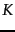. We seek thedocuments of the collection with the highest vector space scores on the given query. We now initiate the study of determining thedocuments with the highest vector space scores for a query. Typically, we seek thesetop documents in ordered by decreasing score; for instance many search engines use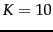to retrieve and rank-order the first page of the ten best results. Here we give the basic algorithm for this computation; we develop a fuller treatment of efficient techniques and approximations in Chapter 7 .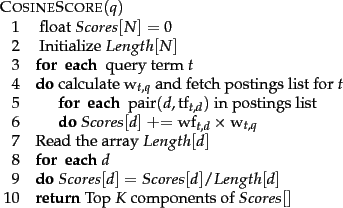Figure 6.14 gives the basic algorithm for computing vector space scores. The array Length holds the lengths (normalization factors) for each of the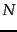documents, whereas the array Scores holds the scores for each of the documents. When the scores are finally computed in Step 9, all that remains in Step 10 is to pick off thedocuments with the highest scores.

The outermost loop beginning Step 3 repeats the updating of Scores, iterating over each query term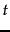in turn. In Step 5 we calculate the weight in the query vector for term. Steps 6-8 update the score of each document by adding in the contribution from term. This process of adding in contributions one query term at a time is sometimes known as term-at-a-time scoring or accumulation, and theelements of the array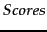are therefore known as accumulators . For this purpose, it would appear necessary to store, with each postings entry, the weight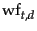of termin document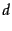(we have thus far used either tf or tf-idf for this weight, but leave open the possibility of other functions to be developed in Section 6.4 ). In fact this is wasteful, since storing this weight may require a floating point number. Two ideas help alleviate this space problem. First, if we are using inverse document frequency , we need not precompute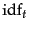; it suffices to store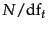at the head of the postings for. Second, we store the term frequency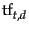for each postings entry. Finally, Step 12 extracts the topscores - this requires a priority queue data structure, often implemented using a heap. Such a heap takes no more than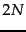comparisons to construct, following which each of thetop scores can be extracted from the heap at a cost of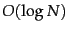comparisons.

Note that the general algorithm of Figure 6.14 does not prescribe a specific implementation of how we traverse the postings lists of the various query terms; we may traverse them one term at a time as in the loop beginning at Step 3, or we could in fact traverse them concurrently as in Figure 1.6 . In such a concurrent postings traversal we compute the scores of one document at a time, so that it is sometimes called document-at-a-time scoring. We will say more about this in Section 7.1.5 .

Exercises.

• If we were to stem jealous and jealousy to a common stem before setting up the vector space, detail how the definitions of tf and idf should be modified.

• Recall the tf-idf weights computed in Exercise 6.2.2. Compute the Euclidean normalized document vectors for each of the documents, where each vector has four components, one for each of the four terms.

• Verify that the sum of the squares of the components of each of the document vectors in Exercise 6.3.3 is 1 (to within rounding error). Why is this the case?

• With term weights as computed in Exercise 6.3.3, rank the three documents by computed score for the query car insurance, for each of the following cases of term weighting in the query:
1. The weight of a term is 1 if present in the query, 0 otherwise.
2. Euclidean normalized idf.Next: Variant tf-idf functions Up: The vector space model Previous: Queries as vectors   Contents   Index